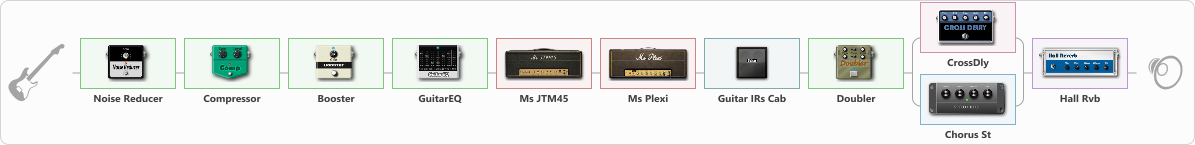# Crunch Strat blue blues

Discussion in 'ToneLib-GFX presets' started by truss van halen, Nov 26, 2020.

1. ### truss van halenWell-Known Member

Crunch Strat blue blues

Preset name: Clrunch truss strat

Effects chain:Effect: "Noise Reducer" (Dynamics / Filter), active - "yes"
{
"Sens" = 100
"Mode" = Hard
}

Effect: "Compressor" (Dynamics / Filter), active - "yes"
{
"Sense" = 31
"Level" = 29
}

Effect: "Booster" (Dynamics / Filter), active - "yes"
{
"Gain" = 67
}

Effect: "GuitarEQ" (Dynamics / Filter), active - "yes"
{
"160 Hz" = 3
"400 Hz" = 1
"800 Hz" = -5
"1.6 kHz" = -3
"3.2 kHz" = 7
"6.4 kHz" = 2
"12 kHz" = 0
"Level (dB)" = 0
}

Effect: "Ms JTM45" (Amp simulators), active - "yes"
{
"Gain" = 50
"Bass" = 58
"Middle" = 59
"Treble" = 38
"Presence" = 50
"Master" = 64
"Level (dB)" = 6
}

Effect: "Ms Plexi" (Amp simulators), active - "yes"
{
"Gain" = 17
"Bass" = 66
"Middle" = 62
"Treble" = 29
"Presence" = 61
"Master" = 67
"Level (dB)" = 5
}

Effect: "Guitar IRs Cab" (Cabinets), active - "yes"
{
"Model" = Fender Twin Rev (2x12")
"Mic Position" = Edge
"Mic Distance" = Near
"Low Cut (Hz)" = 60
"Hi Cut (kHz)" = 20.0
"Mix" = 100
"Level (dB)" = 0
}

Effect: "Doubler" (Dynamics / Filter), active - "yes"
{
"A-Dly" = 75
"A-Inv" = On
"A-Level" = 57
"B-Dly" = 50
"B-Inv" = On
"B-Level" = 34
}

Effect: "Splitter" (Dynamics / Filter), active - "yes"
{
"A-Bypass" = Off
"A-Pan" = 0
"A-Level" = 55
"B-Bypass" = Off
"B-Pan" = 0
"B-Level" = 55

'A' branch:
{

Effect: "CrossDly" (Delay), active - "yes"
{
"Time" = 756
"Feedback" = 53
"Tone" = 80
"Sens" = 38
"Mix" = 43
}
}
'B' branch:
{

Effect: "Chorus St" (Modulation / Sfx), active - "yes"
{
"Speed" = 3.2
"Depth" = 51
"Center" = 6.4
"Mix" = 100
}
}
}

Effect: "Hall Rvb" (Reverberation), active - "yes"
{
"Time" = 9.4
"PreDelay" = 61
"LoDamp" = 49
"HiDamp" = 17
"Mix" = 44
}

Note: You will need to download and install the ToneLib-GFX software to use the preset.

#### Attached Files:

• ###### Crunch_Strat_blue_blues.tlgfx
File size:
1.1 KB
Views:
2,416
Jack Disney and vasia like this.